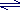# Atom Economy (Atom Utilisation)

## Key Concepts

• One of the key concepts of green chemistry is to reduce waste.
• Waste can be reduced by designing chemical processes to incorporate the maximum amount of all the raw materials into the final product.
• The atom economy (atom utilisation) of a chemical reaction is a measure of the percentage of the starting materials that actually end up in useful products*.
• For the general chemical reaction:
reactantsdesired product + waste products
The atom economy can be calculated in either of two ways:*

 (I) % Atom economy = mass of desired product                 mass of desired product + mass of waste products × 100

 (II) % Atom economy = mass of desired product   total mass of all reactants × 100

Equation (I) is identical to equation (II) because by the Law of Mass Conservation:
total mass of all reactants = mass of desired product + mass of waste products

• The greater the value of the %atom economy, the less the amount of waste product produced.

No ads = no money for us = no free stuff for you!

## Example: Production of Bromoethane

1. Bromoethane (desired product) can be produced from the reactants ethene (ethylene) and hydrogen bromide in an addition reaction.
Calculate the % atom economy for this reaction.

(i) Write the word equation for the reaction :
reactants desired product + waste product
ethene + hydrogen bromide bromoethane
Note: no waste product is formed in this reaction.

(ii) Write the balanced chemical equation for the reaction:
C2H4 + HBr C2H5Br

(iii) Assuming the reaction goes to completion, if 1 mole of each reactant is used, then 1 mole of the product will be produced
1 mole + 1 mole 1 mole

(iv) Calculate the mass of each reactant and product assuming there is a 100% yield
(mass = moles × molar mass)
C2H4
moles = 1 mol
molar mass = (2x12+4x1) = 28 g mol-1
mass =1x28 = 28 g
HBr
moles = 1 mol
molar mass = (1+79.9) = 80.9 g mol-1
mass =1x80.9 = 80.9 g
C2H5Br
moles = 1 mol
molar mass = (2x12+5x1+79.9) = 108.9 g mol-1
mass =1x108.9 = 108.9 g

(v) Calculate the % atom economy:
(a) using equation (I)
 % Atom economy = mass of desired product               mass of desired product + mass of waste products × 100
 % Atom economy = 108.9 g   108.9 g + 0 x 100 = 100%
(b) or using equation (II)
 % Atom economy = mass of desired product total mass of all reactants × 100
 % Atom economy = 108.9 g   28 g + 80.9 g × 100 = 100%

(vi) Conclusion

If no waste product is formed, the reaction goes to completion and the yield of product is 100%, then the % atom economy is 100%.

All the atoms present in the reacting molecules are converted into the desired product.

2. Bromoethane (desired product) and hydrogen bromide (waste product) can be prepared using the reactants ethane and bromine in a substitution reaction.
Calculate the % atom economy for this reaction.

(i) Write the word equation for the reaction :
reactants desired product + waste product
ethane + bromine bromoethane + hydrogen bromide

(ii) Write the balanced chemical equation for the reaction:
C2H6 + Br2 C2H5Br + HBr

(iii) Assuming the reaction goes to completion, if 1 mole of each reactant is used, then 1 mole of the product will be produced
1 mole + 1 mole 1 mole + 1 mole

(iv) Calculate the mass of each reactant and product assuming there is a 100% yield
(mass = moles × molar mass)
C2H6
moles = 1 mol
molar mass = (2x12+6x1) = 30 g mol-1
mass =1x30 = 30 g
Br2
moles = 1 mol
molar mass = (2x79.9) = 159.8 g mol-1
mass =1x159.8 = 159.8 g
C2H5Br
moles = 1 mol
molar mass = (2x12+5x1+79.9) = 108.9 g mol-1
mass =1x108.9 = 108.9 g
HBr
moles = 1
molar mass = (1+79.9) = 80.9 g mol-1
mass =1x80.9 = 80.9 g

(v) Calculate the % atom economy:
(a) using equation (I)
 % Atom economy = mass of desired product               mass of desired product + mass of waste products × 100
 % Atom economy = 108.9 g       108.9 g + 80.9 g × 100 = 57.4%
(b) or using equation (II)
 % Atom economy = mass of desired product   total mass of all reactants × 100
 % Atom economy = 108.9 g       30 g + 159.8 g × 100 = 57.4%

(vi) Conclusion

If one or more waste products are formed, the % atom economy is less than 100%.

Some of the atoms present in the reacting molecules are converted into the desired product, the rest go into the formation of waste products.

Do you know understand this?

Take the test now!

*% Atom economy is not the same as % yield